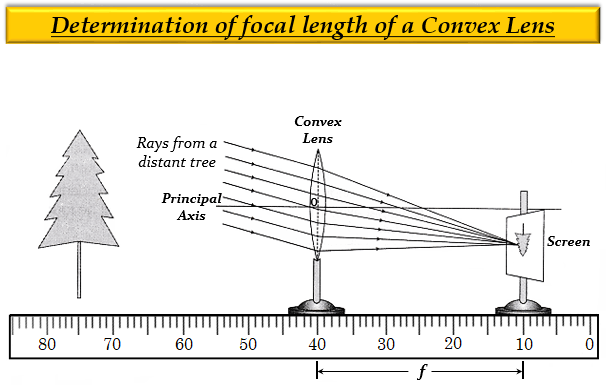# How could you find the focal length of a convex lens rapidly but approximately?

To determine the focal length of a convex lens rapidly but approximately, place the convex lens in a holder (or stand) and keep it in front of a distant object like a tree so that the light rays coming from the tree pass through it. A cardboard screen is put behind the lens. Now, change the distance of the screen from the convex lens until a clear, inverted image of the tree is formed on the screen. Measure the distance of the screen from the lens with the help of a scale. This distance will be the focal length of the convex lens. Here, we have used the fact that the image of an object at a far distance (or, infinity) is formed at the focus of a convex lens, i.e, the distance of the image is equal to the focal length of the lens.

Image is posted for reference onlyUpdated on: 10-Oct-2022

82 Views